# Count Values in Pandas Dataframe

• Last Updated : 09 Aug, 2021

In this article, we are going to count values in Pandas dataframe. First, we will create a data frame, and then we will count the values of different attributes.

Syntax: DataFrame.count(axis=0, level=None, numeric_only=False)

Parameters:

• axis {0 or ‘index’, 1 or ‘columns’}: default 0 Counts are generated for each column if axis=0 or axis=’index’ and counts are generated for each row if axis=1 or axis=”columns”.
• level (nt or str, optional): If the axis is a MultiIndex, count along a particular level, collapsing into a DataFrame. A str specifies the level name.
• numeric_only (boolean, default False): It includes only int, float or boolean value.

Returns: It returns count of non-null values and if level is used it returns dataframe

Step-by-step approach:

Step 1: Importing libraries.

## Python3

 `# importing libraries``import` `numpy as np``import` `pandas as pd`

Step 2: Creating Dataframe

## Python3

 `# Creating dataframe with``# some missing values``NaN ``=` `np.nan``dataframe ``=` `pd.DataFrame({``'Name'``: [``'Shobhit'``, ``'Vaibhav'``,``                                   ``'Vimal'``, ``'Sourabh'``,``                                   ``'Rahul'``, ``'Shobhit'``],``                          ``'Physics'``: [``11``, ``12``, ``13``, ``14``, NaN, ``11``],``                          ``'Chemistry'``: [``10``, ``14``, NaN, ``18``, ``20``, ``10``],``                          ``'Math'``: [``13``, ``10``, ``15``, NaN, NaN, ``13``]})` `display(dataframe)`

Output: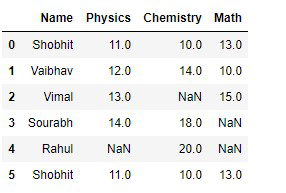Created Dataframe

Step 3: In this step, we just simply use the .count() function to count all the values of different columns.

## Python3

 `# using dataframe.count()``# to count all values``dataframe.count()`

Output: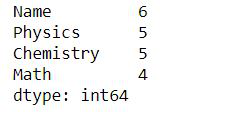We can see that there is a difference in count value as we have missing values. There are 5 values in the Name column,4 in Physics and Chemistry, and 3 in Math. In this case, it uses it’s an argument with its default values.

Step 4: If we want to count all the values with respect to row then we have to pass axis=1 or ‘columns’.

## Python3

 `# we can pass either axis=1 or``# axos='columns' to count with respect to row``print``(dataframe.count(axis ``=` `1``))` `print``(dataframe.count(axis ``=` `'columns'``))`

Output: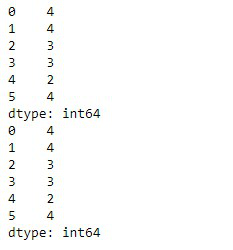count with respect to row

Step 5: Now if we want to count null values in our dataframe.

## Python3

 `# it will give the count``# of individual columns count of null values``print``(dataframe.isnull().``sum``())` `# it will give the total null``# values present in our dataframe``print``(``"Total Null values count: "``,``      ``dataframe.isnull().``sum``().``sum``())`

Output: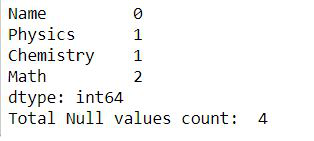Step 6:. Some examples to use .count()

Now we want to count no of students whose physics marks are greater than 11.

## Python3

 `# count of student with greater``# than 11 marks in physics``print``(``"Count of students with physics marks greater than 11 is->"``,``      ``dataframe[dataframe[``'Physics'``] > ``11``][``'Name'``].count())` `# resultant of above dataframe``dataframe[dataframe[``'Physics'``]>``11``]`

Output: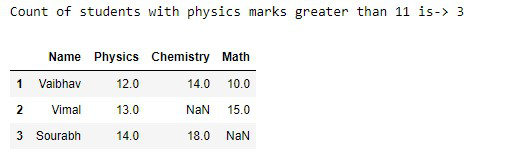Physics>11

Count of students whose physics marks are greater than 10,chemistry marks are greater than 11 and math marks are greater than 9.

## Python3

 `# Count of students whose physics marks``# are greater than 10,chemistry marks are``# greater than 11 and math marks are greater than 9.``print``(``"Count of students ->"``,``      ``dataframe[(dataframe[``'Physics'``] > ``10``) &``                ``(dataframe[``'Chemistry'``] > ``11``) &``                ``(dataframe[``'Math'``] > ``9``)][``'Name'``].count())` `# dataframe of above result``dataframe[(dataframe[``'Physics'``] > ``10` `) &``          ``(dataframe[``'Chemistry'``] > ``11` `) &``          ``(dataframe[``'Math'``] > ``9` `)]`

Output: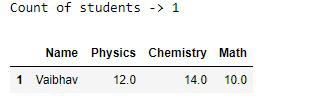Physics>10 ,Chemistry>11,Maths>9

Below is the full implementation:

## Python3

 `# importing Libraries``import` `pandas as pd``import` `numpy as np` `# Creating dataframe using dictionary``NaN ``=` `np.nan``dataframe ``=` `pd.DataFrame({``'Name'``: [``'Shobhit'``, ``'Vaibhav'``,``                                   ``'Vimal'``, ``'Sourabh'``,``                                   ``'Rahul'``, ``'Shobhit'``],``                          ``'Physics'``: [``11``, ``12``, ``13``, ``14``, NaN, ``11``],``                          ``'Chemistry'``: [``10``, ``14``, NaN, ``18``, ``20``, ``10``],``                          ``'Math'``: [``13``, ``10``, ``15``, NaN, NaN, ``13``]})` `print``(``"Created Dataframe"``)``print``(dataframe)` `# finding Count of all columns``print``(``"Count of all values wrt columns"``)``print``(dataframe.count())` `# Count according to rows``print``(``"Count of all values wrt rows"``)``print``(dataframe.count(axis``=``1``))``print``(dataframe.count(axis``=``'columns'``))` `# count of null values``print``(``"Null Values counts "``)``print``(dataframe.isnull().``sum``())``print``(``"Total null values"``,``      ``dataframe.isnull().``sum``().``sum``())` `# count of student with greater``# than 11 marks in physics``print``(``"Count of students with physics marks greater than 11 is->"``,``      ``dataframe[dataframe[``'Physics'``] > ``11``][``'Name'``].count())` `# resultant of above dataframe``print``(dataframe[dataframe[``'Physics'``] > ``11``])``print``(``"Count of students ->"``,``      ``dataframe[(dataframe[``'Physics'``] > ``10``) &``                ``(dataframe[``'Chemistry'``] > ``11``) &``                ``(dataframe[``'Math'``] > ``9``)][``'Name'``].count())` `print``(dataframe[(dataframe[``'Physics'``] > ``10``) &``                ``(dataframe[``'Chemistry'``] > ``11``) &``                ``(dataframe[``'Math'``] > ``9``)])`

Output: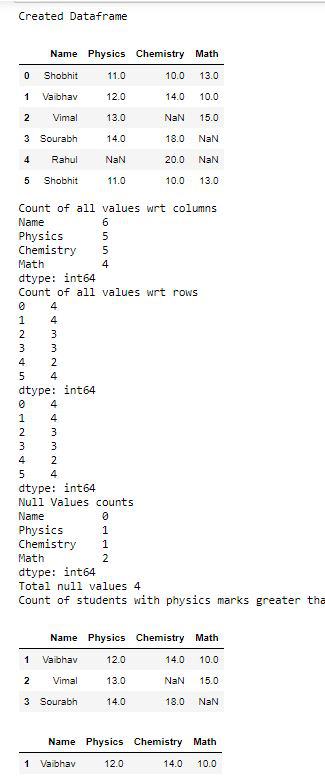My Personal Notes arrow_drop_up